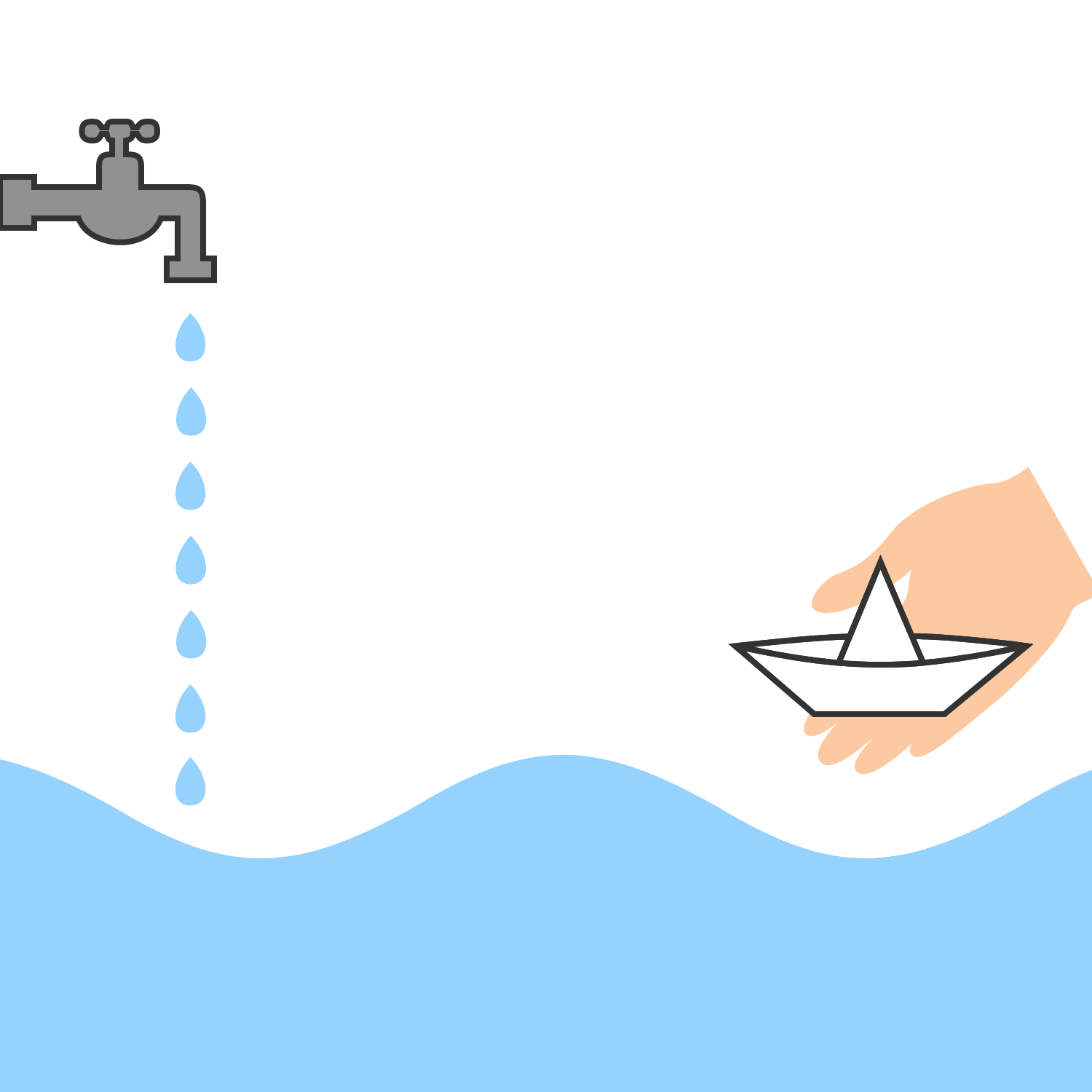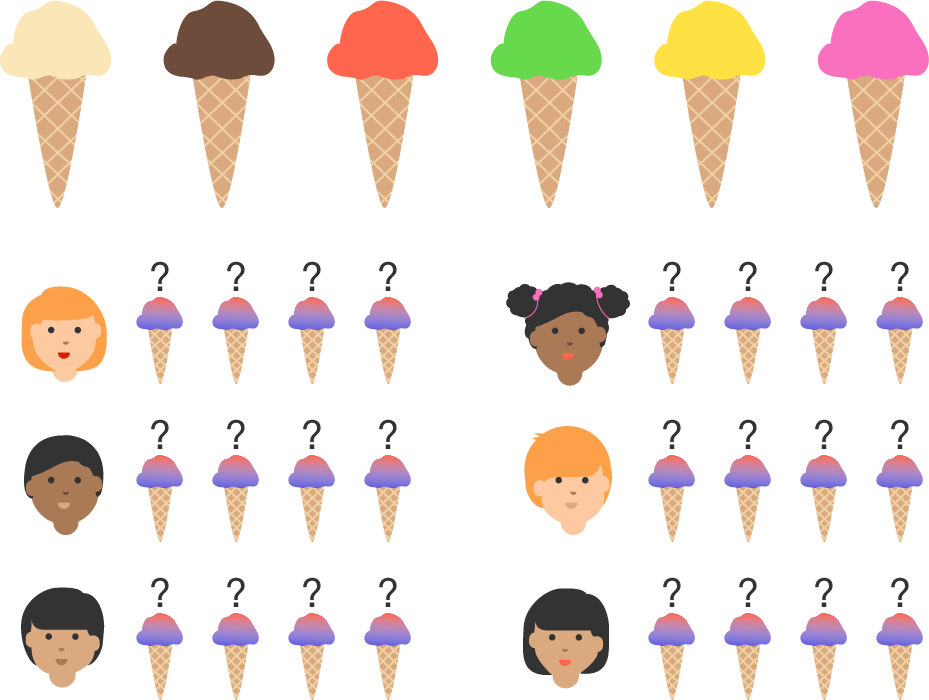# Problems of the Week

Contribute a problem

# 2017-08-07 Basic

$\Large 0 = 1\ \square\ 2\ \square\ 3\ \square\ 4\ \square\ 5\ \square\ 6\ \square\ 7\ \square\ 8\ \square\ 9$

Can you fill the boxes with $+$ and $-$ to make this equation true?

$6$ is divisible by $6$.
$6\times6$ is divisible by $6+6$.
$6\times6\times6$ is divisible by $6+6+6$.

Is it true that $\underbrace{6\times6\times\cdots\times6}_{n\text{ times}}$ is divisible by $\underbrace{6+6+\cdots+6}_{n\text{ times}}$ for all positive integers $n$?The drops from a leaky faucet form a steady wave in the water, a snapshot of which is shown in the diagram above.

Rob carefully lowers his paper boat into the water such that it starts at the bottom of the wave.

True or False?

Rob's boat will move away from the faucet along with the ripples.

$\large \begin{matrix} &&& & & \square \\ &&& & \square & \square \\ + & & & \square & \square & \square \\ \hline \\ & & 1 & 1 & 1 & 1 \end{matrix}$

Each box contains a non-zero digit, not necessarily distinct from other numbers. Is the equation above possible?The store has 6 flavors of ice-cream. Each of 6 friends likes exactly 4 flavors.

Is there guaranteed to be a flavor which at least 4 friends like?

×

Problem Loading...

Note Loading...

Set Loading...